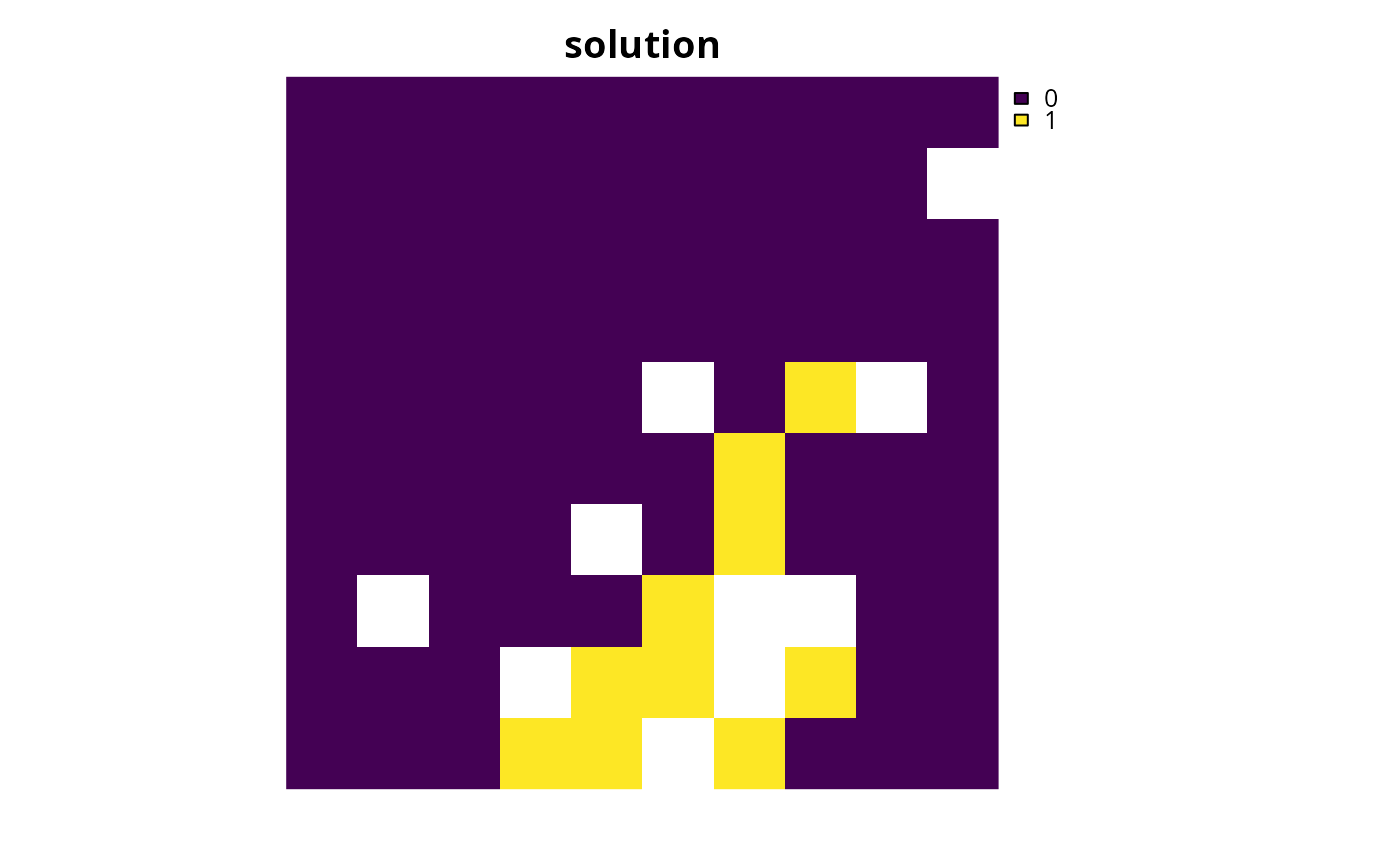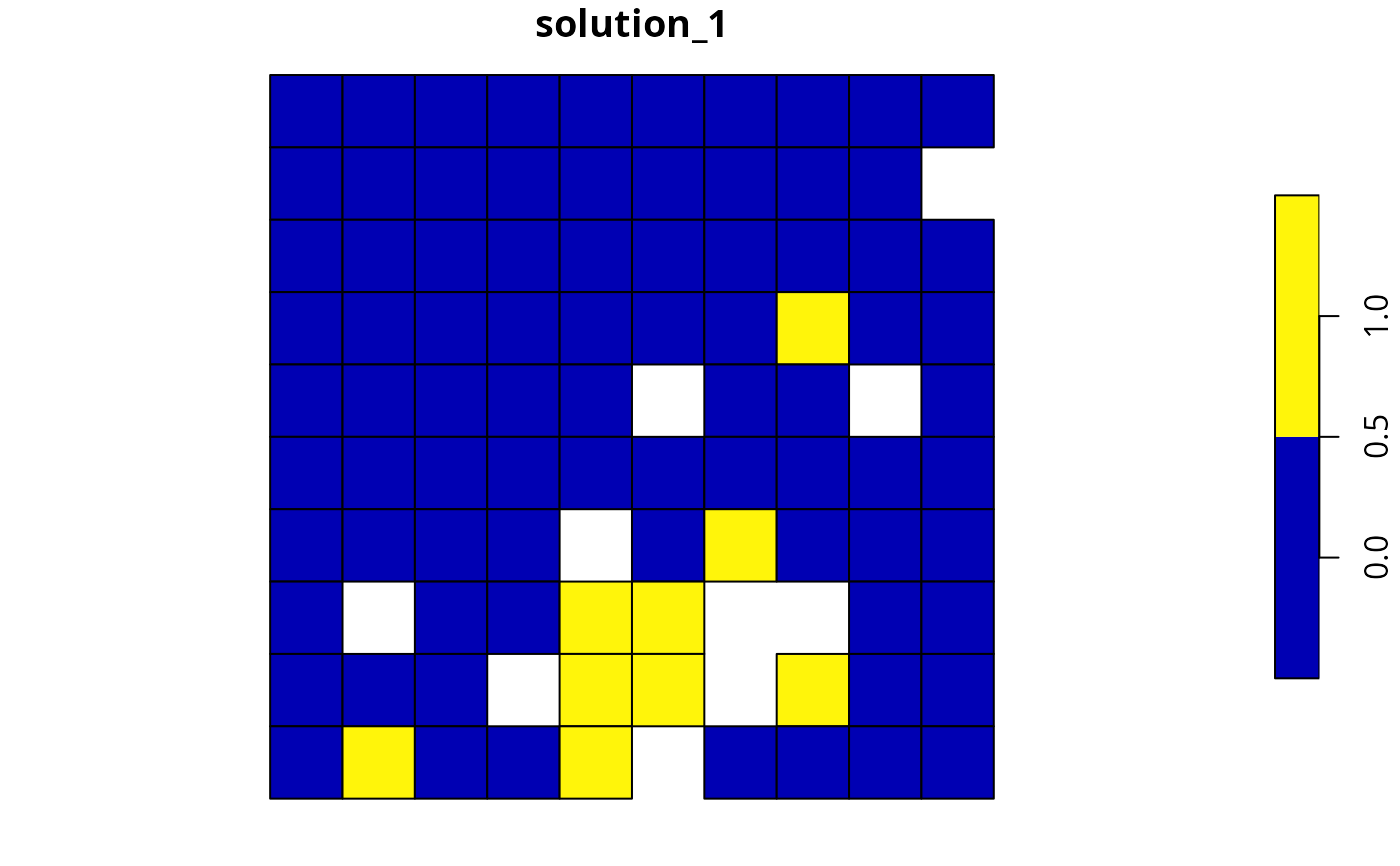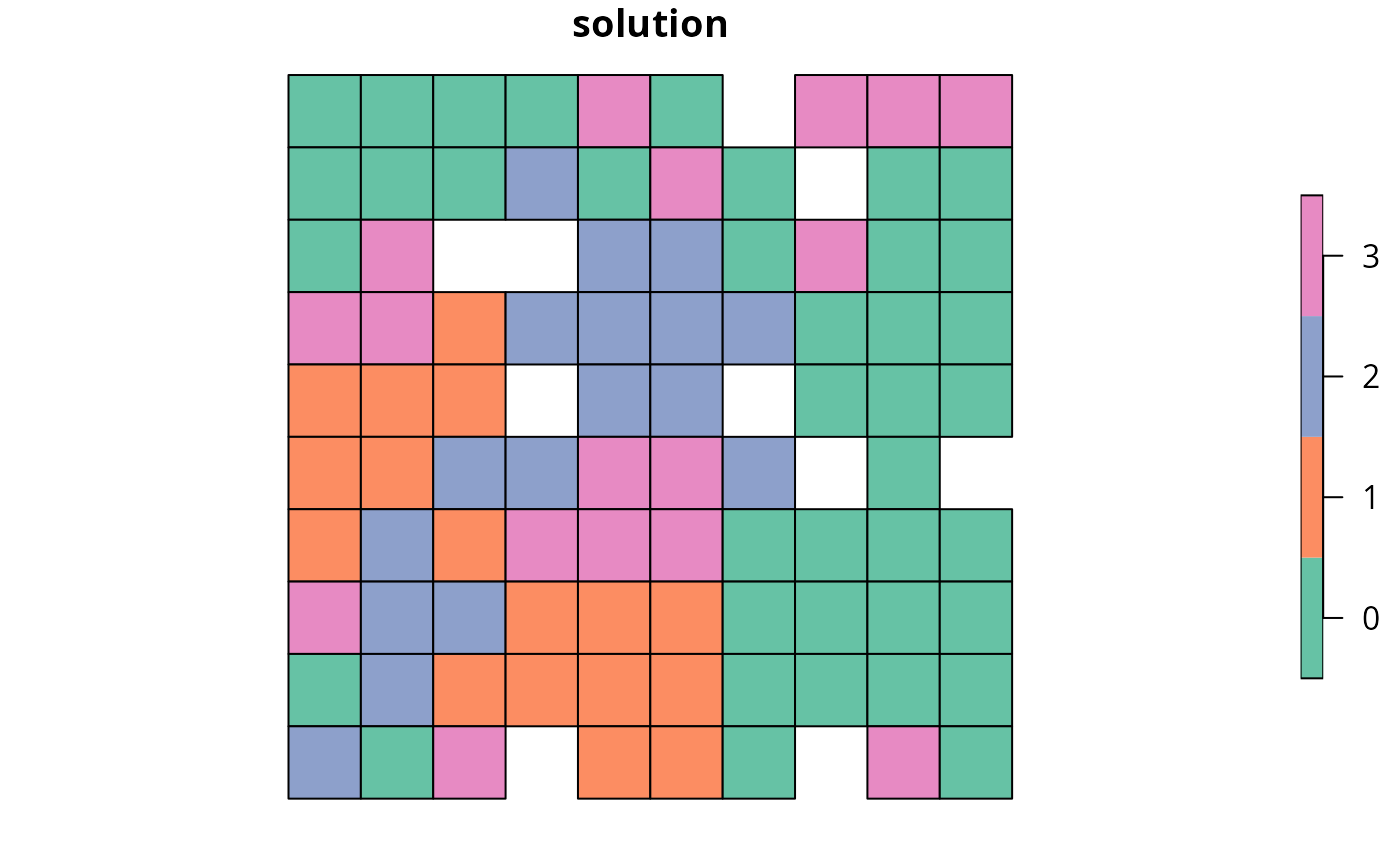Calculate how well feature representation targets are met by a solution to a conservation planning problem. It is useful for understanding if features are adequately represented by a solution. Note that this function can only be used with problems that contain targets.

## Usage

eval_target_coverage_summary(
x,
solution,
include_zone = number_of_zones(x) > 1,
include_sense = number_of_zones(x) > 1
)

## Arguments

x

problem() object.

solution

numeric, matrix, data.frame, terra::rast(), or sf::sf() object. The argument should be in the same format as the planning unit cost data in the argument to x. See the Solution format section for more information.

include_zone

logical include the zone column in the output? Defaults to TRUE for problems that contain multiple zones.

include_sense

logical include the sense column in the output? Defaults to TRUE for problems that contain multiple zones.

## Value

A tibble::tibble() object. Here, each row describes information for a different target. It contains the following columns:

feature

character name of the feature associated with each target.

zone

list of character zone names associated with each target. This column is in a list-column format because a single target can correspond to multiple zones (see add_manual_targets() for details and examples). For an example of converting the list-column format to a standard character column format, please see the Examples section. This column is only included if the argument to include_zones is TRUE.

sense

character sense associated with each target. Sense values specify the nature of the target. Typically (e.g., when using the add_absolute_targets() or add_relative_targets() functions), targets are specified using sense values indicating that the total amount of a feature held within a solution (ideally) be greater than or equal to a threshold amount (i.e., a sense value of ">="). Additionally, targets (i.e., using the add_manual_targets() function) can also be specified using sense values indicating that the total amount of a feature held within a solution must be equal to a threshold amount (i.e., a sense value of "=") or smaller than or equal to a threshold amount (i.e., a sense value of "<="). This column is only included if the argument to include_sense is TRUE.

total_amount

numeric total amount of the feature available across the entire conservation planning problem for meeting each target (not just planning units selected within the solution). For problems involving a single zone, this column is calculated as the sum of all of the values for a given feature (similar to values in the total_amount column produced by the eval_feature_representation_summary() function). For problems involving multiple zones, this column is calculated as the sum of the values for the feature associated with target (per the "feature" column), across the zones associated with the target (per the "zone" column).

absolute_target

numeric total threshold amount associated with each target.

absolute_held

numeric total amount held within the solution for the feature and (if relevant) zones associated with each target (per the "feature" and "zone" columns, respectively). This column is calculated as the sum of the feature data, supplied when creating a problem() object (e.g., presence/absence values), weighted by the status of each planning unit in the solution (e.g., selected or not for prioritization).

absolute_shortfall

numeric total amount by which the solution fails to meet each target. This column is calculated as the difference between the total amount held within the solution for the feature and (if relevant) zones associated with the target (i.e., "absolute_held" column) and the target total threshold amount (i.e., "absolute_target" column), with values set to zero depending on the sense specified for the target (e.g., if the target sense is >= then the difference is set to zero if the value in the "absolute_held" is smaller than that in the "absolute_target" column).

relative_target

numeric proportion threshold amount associated with each target. This column is calculated by dividing the total threshold amount associated with each target (i.e., "absolute_target" column) by the total amount associated with each target (i.e., "total_amount" column).

relative_held

numeric proportion held within the solution for the feature and (if relevant) zones associated with each target (per the "feature" and "zone" columns, respectively). This column is calculated by dividing the total amount held for each target (i.e., "absolute_held" column) by the total amount for with each target (i.e., "total_amount" column).

relative_shortfall

numeric proportion by which the solution fails to meet each target. This column is calculated by dividing the total shortfall for each target (i.e., "absolute_shortfall" column) by the total amount for each target (i.e., "total_amount" column).

met

logical indicating if each target is met by the solution. This column is calculated by checking if the total shortfall associated with each target (i.e., "absolute_shortfall" column) is equal to zero.

## Solution format

Broadly speaking, the argument to solution must be in the same format as the planning unit data in the argument to x. Further details on the correct format are listed separately for each of the different planning unit data formats:

x has numeric planning units

The argument to solution must be a numeric vector with each element corresponding to a different planning unit. It should have the same number of planning units as those in the argument to x. Additionally, any planning units missing cost (NA) values should also have missing (NA) values in the argument to solution.

x has matrix planning units

The argument to solution must be a matrix vector with each row corresponding to a different planning unit, and each column correspond to a different management zone. It should have the same number of planning units and zones as those in the argument to x. Additionally, any planning units missing cost (NA) values for a particular zone should also have a missing (NA) values in the argument to solution.

x has terra::rast() planning units

The argument to solution be a terra::rast() object where different grid cells (pixels) correspond to different planning units and layers correspond to a different management zones. It should have the same dimensionality (rows, columns, layers), resolution, extent, and coordinate reference system as the planning units in the argument to x. Additionally, any planning units missing cost (NA) values for a particular zone should also have missing (NA) values in the argument to solution.

x has data.frame planning units

The argument to solution must be a data.frame with each column corresponding to a different zone, each row corresponding to a different planning unit, and cell values corresponding to the solution value. This means that if a data.frame object containing the solution also contains additional columns, then these columns will need to be subsetted prior to using this function (see below for example with sf::sf() data). Additionally, any planning units missing cost (NA) values for a particular zone should also have missing (NA) values in the argument to solution.

x has sf::sf() planning units

The argument to solution must be a sf::sf() object with each column corresponding to a different zone, each row corresponding to a different planning unit, and cell values corresponding to the solution value. This means that if the sf::sf() object containing the solution also contains additional columns, then these columns will need to be subsetted prior to using this function (see below for example). Additionally, the argument to solution must also have the same coordinate reference system as the planning unit data. Furthermore, any planning units missing cost (NA) values for a particular zone should also have missing (NA) values in the argument to solution.

See summaries for an overview of all functions for summarizing solutions.

Other summaries: eval_asym_connectivity_summary(), eval_boundary_summary(), eval_connectivity_summary(), eval_cost_summary(), eval_feature_representation_summary(), eval_n_summary()

## Examples

# \dontrun{
# set seed for reproducibility
set.seed(500)

sim_pu_raster <- get_sim_pu_raster()
sim_pu_polygons <- get_sim_pu_polygons()
sim_features <- get_sim_features()
sim_zones_pu_polygons <- get_sim_zones_pu_polygons()
sim_zones_features <- get_sim_zones_features()

# build minimal conservation problem with raster data
p1 <-
problem(sim_pu_raster, sim_features) %>%

# solve the problem
s1 <- solve(p1)

# print solution
print(s1)
#> class       : SpatRaster
#> dimensions  : 10, 10, 1  (nrow, ncol, nlyr)
#> resolution  : 0.1, 0.1  (x, y)
#> extent      : 0, 1, 0, 1  (xmin, xmax, ymin, ymax)
#> coord. ref. : Undefined Cartesian SRS
#> source(s)   : memory
#> varname     : sim_pu_raster
#> name        : layer
#> min value   :     0
#> max value   :     1

# plot solution
plot(s1, main = "solution", axes = FALSE)# calculate target coverage by the solution
r1 <- eval_target_coverage_summary(p1, s1)
print(r1, width = Inf) # note: width = Inf tells R to print all columns
#> # A tibble: 5 × 9
#>   feature   met   total_amount absolute_target absolute_held absolute_shortfall
#>   <chr>     <lgl>        <dbl>           <dbl>         <dbl>              <dbl>
#> 1 feature_1 TRUE          83.3            8.33          8.91                  0
#> 2 feature_2 TRUE          31.2            3.12          3.13                  0
#> 3 feature_3 TRUE          72.0            7.20          7.34                  0
#> 4 feature_4 TRUE          42.7            4.27          4.35                  0
#> 5 feature_5 TRUE          56.7            5.67          6.01                  0
#>   relative_target relative_held relative_shortfall
#>             <dbl>         <dbl>              <dbl>
#> 1             0.1         0.107                  0
#> 2             0.1         0.100                  0
#> 3             0.1         0.102                  0
#> 4             0.1         0.102                  0
#> 5             0.1         0.106                  0

# build minimal conservation problem with polygon data
p2 <-
problem(sim_pu_polygons, sim_features, cost_column = "cost") %>%

# solve the problem
s2 <- solve(p2)

# print first six rows of the attribute table
#> Simple feature collection with 6 features and 4 fields
#> Geometry type: POLYGON
#> Dimension:     XY
#> Bounding box:  xmin: 0 ymin: 0.9 xmax: 0.6 ymax: 1
#> Projected CRS: Undefined Cartesian SRS
#> # A tibble: 6 × 5
#>    cost locked_in locked_out solution_1                                     geom
#>   <dbl> <lgl>     <lgl>           <dbl>                            <POLYGON [m]>
#> 1  216. FALSE     FALSE               0      ((0 1, 0.1 1, 0.1 0.9, 0 0.9, 0 1))
#> 2  213. FALSE     FALSE               0 ((0.1 1, 0.2 1, 0.2 0.9, 0.1 0.9, 0.1 1…
#> 3  207. FALSE     FALSE               0 ((0.2 1, 0.3 1, 0.3 0.9, 0.2 0.9, 0.2 1…
#> 4  209. FALSE     TRUE                0 ((0.3 1, 0.4 1, 0.4 0.9, 0.3 0.9, 0.3 1…
#> 5  214. FALSE     FALSE               0 ((0.4 1, 0.5 1, 0.5 0.9, 0.4 0.9, 0.4 1…
#> 6  214. FALSE     FALSE               0 ((0.5 1, 0.6 1, 0.6 0.9, 0.5 0.9, 0.5 1…

# plot solution
plot(s2[, "solution_1"])# calculate target coverage by the solution
r2 <- eval_target_coverage_summary(p2, s2[, "solution_1"])
print(r2, width = Inf)
#> # A tibble: 5 × 9
#>   feature   met   total_amount absolute_target absolute_held absolute_shortfall
#>   <chr>     <lgl>        <dbl>           <dbl>         <dbl>              <dbl>
#> 1 feature_1 TRUE          74.5            7.45          8.05                  0
#> 2 feature_2 TRUE          28.1            2.81          2.83                  0
#> 3 feature_3 TRUE          64.9            6.49          6.65                  0
#> 4 feature_4 TRUE          38.2            3.82          3.87                  0
#> 5 feature_5 TRUE          50.7            5.07          5.41                  0
#>   relative_target relative_held relative_shortfall
#>             <dbl>         <dbl>              <dbl>
#> 1             0.1         0.108                  0
#> 2             0.1         0.101                  0
#> 3             0.1         0.103                  0
#> 4             0.1         0.101                  0
#> 5             0.1         0.107                  0

# build multi-zone conservation problem with polygon data
p3 <-
problem(
sim_zones_pu_polygons, sim_zones_features,
cost_column = c("cost_1", "cost_2", "cost_3")
) %>%
add_relative_targets(matrix(runif(15, 0.1, 0.2), nrow = 5, ncol = 3)) %>%

# solve the problem
s3 <- solve(p3)

# print solution
print(s3)
#> Simple feature collection with 90 features and 9 fields
#> Geometry type: POLYGON
#> Dimension:     XY
#> Bounding box:  xmin: 0 ymin: 0 xmax: 1 ymax: 1
#> Projected CRS: Undefined Cartesian SRS
#> # A tibble: 90 × 10
#>    cost_1 cost_2 cost_3 locked_1 locked_2 locked_3 solution_1_zone_1
#>     <dbl>  <dbl>  <dbl> <lgl>    <lgl>    <lgl>                <dbl>
#>  1   216.   183.   205. FALSE    FALSE    FALSE                    0
#>  2   213.   189.   210. FALSE    FALSE    FALSE                    0
#>  3   207.   194.   215. TRUE     FALSE    FALSE                    0
#>  4   209.   198.   219. FALSE    FALSE    FALSE                    0
#>  5   214.   200.   221. FALSE    FALSE    FALSE                    0
#>  6   214.   203.   225. FALSE    FALSE    FALSE                    0
#>  7   211.   209.   223. FALSE    FALSE    FALSE                    0
#>  8   210.   212.   222. TRUE     FALSE    FALSE                    0
#>  9   204.   218.   214. FALSE    FALSE    FALSE                    0
#> 10   213.   183.   206. FALSE    FALSE    FALSE                    0
#> # ℹ 80 more rows
#> # ℹ 3 more variables: solution_1_zone_2 <dbl>, solution_1_zone_3 <dbl>,
#> #   geom <POLYGON [m]>

# create new column representing the zone id that each planning unit
# was allocated to in the solution
s3$solution <- category_vector( s3[, c("solution_1_zone_1", "solution_1_zone_2", "solution_1_zone_3")] ) s3$solution <- factor(s3$solution) # plot solution plot(s3[, "solution"])# calculate target coverage by the solution r3 <- eval_target_coverage_summary( p3, s3[, c("solution_1_zone_1", "solution_1_zone_2", "solution_1_zone_3")] ) print(r3, width = Inf) #> # A tibble: 15 × 11 #> feature zone sense met total_amount absolute_target absolute_held #> <chr> <list> <chr> <lgl> <dbl> <dbl> <dbl> #> 1 feature_1 <chr > >= TRUE 75.1 13.8 14.9 #> 2 feature_2 <chr > >= TRUE 28.0 4.82 5.14 #> 3 feature_3 <chr > >= TRUE 65.0 12.8 12.8 #> 4 feature_4 <chr > >= TRUE 38.0 5.58 6.64 #> 5 feature_5 <chr > >= TRUE 51.2 9.27 9.96 #> 6 feature_1 <chr > >= TRUE 75.1 9.06 15.0 #> 7 feature_2 <chr > >= TRUE 28.0 4.23 5.58 #> 8 feature_3 <chr > >= TRUE 65.0 12.5 13.6 #> 9 feature_4 <chr > >= TRUE 38.0 6.96 7.14 #> 10 feature_5 <chr > >= TRUE 51.2 8.76 9.96 #> 11 feature_1 <chr > >= TRUE 75.1 9.63 13.3 #> 12 feature_2 <chr > >= TRUE 28.0 5.29 5.65 #> 13 feature_3 <chr > >= TRUE 65.0 11.5 11.8 #> 14 feature_4 <chr > >= TRUE 38.0 4.43 7.71 #> 15 feature_5 <chr > >= TRUE 51.2 8.86 9.04 #> absolute_shortfall relative_target relative_held relative_shortfall #> <dbl> <dbl> <dbl> <dbl> #> 1 0 0.183 0.198 0 #> 2 0 0.173 0.184 0 #> 3 0 0.198 0.198 0 #> 4 0 0.147 0.175 0 #> 5 0 0.181 0.195 0 #> 6 0 0.121 0.200 0 #> 7 0 0.151 0.200 0 #> 8 0 0.193 0.210 0 #> 9 0 0.183 0.188 0 #> 10 0 0.171 0.195 0 #> 11 0 0.128 0.177 0 #> 12 0 0.189 0.202 0 #> 13 0 0.176 0.181 0 #> 14 0 0.116 0.203 0 #> 15 0 0.173 0.177 0 # create a new column with character values containing the zone names, # by extracting these data out of the zone column # (which is in list-column format) r3$zone2 <- vapply(r3\$zone, FUN.VALUE = character(1), paste, sep = " & ")

# print r3 again to show the new column
print(r3, width = Inf)
#> # A tibble: 15 × 12
#>    feature   zone      sense met   total_amount absolute_target absolute_held
#>    <chr>     <list>    <chr> <lgl>        <dbl>           <dbl>         <dbl>
#>  1 feature_1 <chr > >=    TRUE          75.1           13.8          14.9
#>  2 feature_2 <chr > >=    TRUE          28.0            4.82          5.14
#>  3 feature_3 <chr > >=    TRUE          65.0           12.8          12.8
#>  4 feature_4 <chr > >=    TRUE          38.0            5.58          6.64
#>  5 feature_5 <chr > >=    TRUE          51.2            9.27          9.96
#>  6 feature_1 <chr > >=    TRUE          75.1            9.06         15.0
#>  7 feature_2 <chr > >=    TRUE          28.0            4.23          5.58
#>  8 feature_3 <chr > >=    TRUE          65.0           12.5          13.6
#>  9 feature_4 <chr > >=    TRUE          38.0            6.96          7.14
#> 10 feature_5 <chr > >=    TRUE          51.2            8.76          9.96
#> 11 feature_1 <chr > >=    TRUE          75.1            9.63         13.3
#> 12 feature_2 <chr > >=    TRUE          28.0            5.29          5.65
#> 13 feature_3 <chr > >=    TRUE          65.0           11.5          11.8
#> 14 feature_4 <chr > >=    TRUE          38.0            4.43          7.71
#> 15 feature_5 <chr > >=    TRUE          51.2            8.86          9.04
#>    absolute_shortfall relative_target relative_held relative_shortfall zone2
#>                 <dbl>           <dbl>         <dbl>              <dbl> <chr>
#>  1                  0           0.183         0.198                  0 zone_1
#>  2                  0           0.173         0.184                  0 zone_1
#>  3                  0           0.198         0.198                  0 zone_1
#>  4                  0           0.147         0.175                  0 zone_1
#>  5                  0           0.181         0.195                  0 zone_1
#>  6                  0           0.121         0.200                  0 zone_2
#>  7                  0           0.151         0.200                  0 zone_2
#>  8                  0           0.193         0.210                  0 zone_2
#>  9                  0           0.183         0.188                  0 zone_2
#> 10                  0           0.171         0.195                  0 zone_2
#> 11                  0           0.128         0.177                  0 zone_3
#> 12                  0           0.189         0.202                  0 zone_3
#> 13                  0           0.176         0.181                  0 zone_3
#> 14                  0           0.116         0.203                  0 zone_3
#> 15                  0           0.173         0.177                  0 zone_3
# }